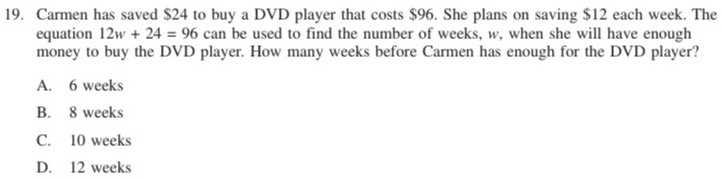### ¿Todavía tienes preguntas de matemáticas?

Pregunte a nuestros tutores expertos
Algebra
Pregunta19. Carmen has saved $$\ 24$$ to buy a DVD player that costs $$\ 96$$ . She plans on saving $$\ 12$$ each week. The equation $$12 w + 24 = 96$$ can be used to find the number of weeks, $$w$$ , when she will have enough money to buy the DVD player. How many weeks before Carmen has enough for the DVD player? A. $$6$$ weeks B. $$8$$ weeks C. $$10$$ weeks D. $$12$$ weeks